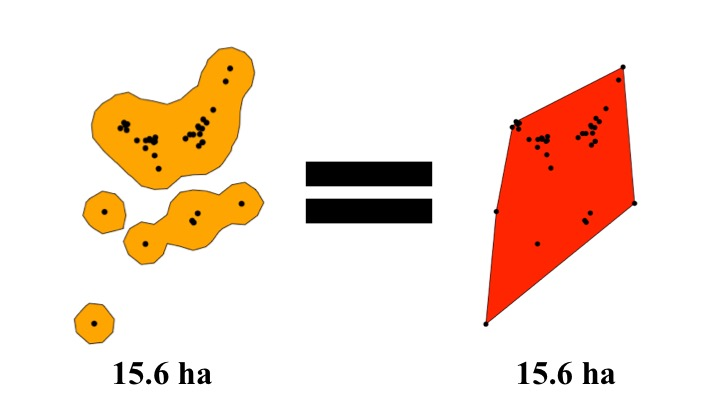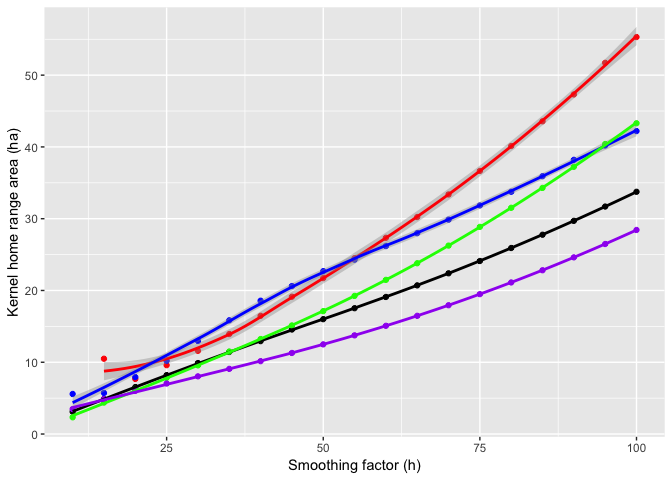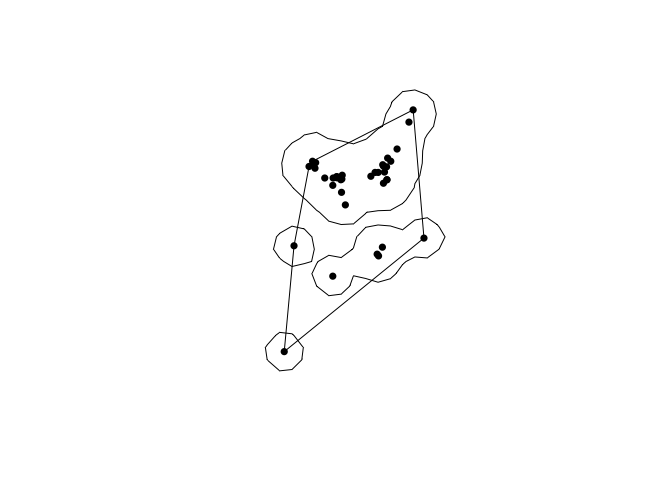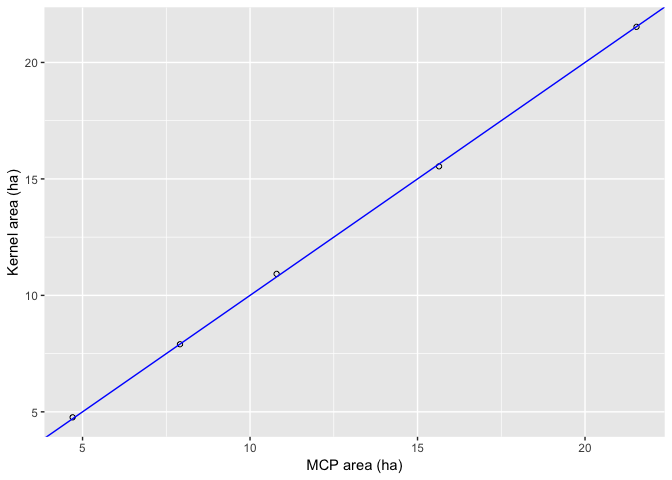Kernel density estimators are often used for measuring home ranges and this is useful for measuring interactions between animals and their environment. However, kernels are sensitive to the choice of a smoothing factor (h). Home range size (using the 95% contour line) increases with the smoothing factor, but the rate of increase depends on the distribution of relocations so the relationship is inconsistent between individuals. For example, notice in the plot below for 5 animals how the relationship between smoothing factor and home range area differs between individuals and isn’t always linear.For reptiles, the choice of h is even more difficult because telemetry studies typically have few relocations (< 50 per animal) compared to studies on larger animals with GPS collars. Further, habitat use in reptiles is typically clustered at a few core areas where animals spend lots of time. Row & Blouin-Demers (2006) recommended using an h that creates a 95% contour area equal to the 100% minimum convex polygon.

This post will be helpful if you:

• Tracked reptiles (or another animal with a low number of relocations) and are quantifying habitat use,
• Want to make your 95% kernel area equal to the 100% minimum convex polygon for each individual.

As in previous posts, I will use the `adehabitatHR` package on sample data to show how the functions work. All the code and data used are available on my GitHub page.

First, let’s load some sample data.

``````# Read the csv file (should be in your working directory)
stringsAsFactors = FALSE)

# SpatialPointsDataFrame objects don't like missing values
# Remove rows with NA's
tracking.df <- tracking.df[!is.na(tracking.df\$x) & !is.na(tracking.df\$y),]

# Create a copy of the object to make into a SpatialPointsDataFrame
# Only include three columns (id, x, and y coordinates) for estimating home ranges
tracking.sp <- tracking.df

# Create a SpatialPointsDataFrame by defining the coordinates
library(sp)
coordinates(tracking.sp) <- c("x", "y")

# Set the coordinate reference system (CRS)
# https://www.nceas.ucsb.edu/~frazier/RSpatialGuides/OverviewCoordinateReferenceSystems.pdf
# The sample data are UTM points in WGS84 from zone 18N
proj4string(tracking.sp) <- CRS( "+proj=utm +zone=18 +datum=WGS84 +units=m +no_defs" )``````

I have written two functions in R to create kernel home ranges equal in area to the 100% minimum convex polygon. The functions should work on any tracking data set.

1. The first function (`reptile.kd.h.fn`) estimates the required smoothing factor for each individual in a data set.
2. The second function (`reptile.kd.homerange.fn`) uses a vector of smoothing factors to generate 95% contour lines (as a list of spatial polygons) for each individual in a data set.

Load the two functions into the environment. To save space, I haven’t shown the full functions in this post, but the code can be found on my GitHub page.

Now, I can apply the first function to the sample data to get the smoothing factor for each animal.

``````# The sp object should contain at least x,y, & id
# system.time will time how long it takes. The function is slow for large data sets.
system.time(h.pred <- reptile.kd.h.fn(tracking.sp))  ``````
``````##  24.04039
##  49.64365
##  34.55761
##  33.35622
##  14.5727``````
``````##    user  system elapsed
##  14.731   1.205  18.801``````
``````# Takes: 10 - 15 sec for 5 animals

h.pred``````
``##  24.04039 49.64365 34.55761 33.35622 14.57270``

Now the smoothing factors we need are stored in the object `h.pred`. This function is slow for large data sets because it creates kernels and extracts the home range size of the 95% contour line for smoothing factors between 10 and the reference bandwidth for each animal.

The next step is to take these smoothing factors and use them to create home range polygons with the second function.

``````# Calculate kernel home ranges
system.time(test.homeranges <- reptile.kd.homerange.fn(tracking.sp, h.pred))``````
``````##    user  system elapsed
##   0.389   0.015   0.580``````

The home ranges are stored in a list (`test.homeranges`), with each item being a spatial polygon home range with the 95% contour line equalling the area of the 100% minimum convex polygon.How close did we get to our target? We can test this by plotting the areas of the kernel home ranges versus the areas of the minimum convex polygons.Since all points fall very close to the line with a y-intercept of 0 and a slope of 1 (the blue line), the kernel area equals the 100% minimum convex polygon for each of the 5 sample animals.

I hope you find these functions helpful. If the functions are not working for your data, feel free to get in touch and I can try to help troubleshoot.